### SPR-Pages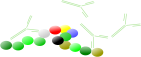back
Quiz
back
back

## Sensorgram quiz

Please read the tutorial before making the quiz. All the answers are 'hidden' in the text. After submitting the quiz you will see your results and a comparison with the other results. The quiz has 30 questions and will take 5 - 10 minutes to complete. Read the questions carefully.
You can make the quiz as often as you like.
Success!

Question 1: Exponential curves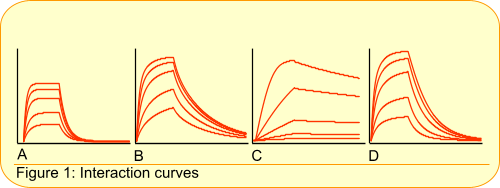Which sensorgram has exponential interaction curves?

A     B     C     D

Question 2: Mass transfer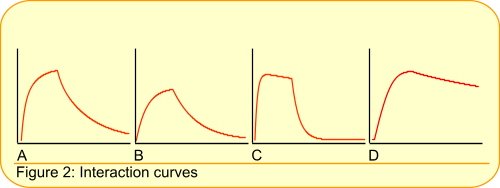Which curve is an exponential interaction curve with mass-transfer?

A     B     C     D

Question 3: Exponential interaction curveWhich sensorgram does not have exponential interaction curves?

A     B     C     D

Question 4: Sensorgram issues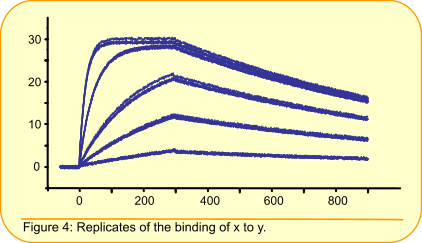What is wrong with this sensorgram?

A: the axis legends are missing
B: there are no replicates
C: the bulk effect is too big
D: not all curves go to steady state

Question 5: Sensorgram issues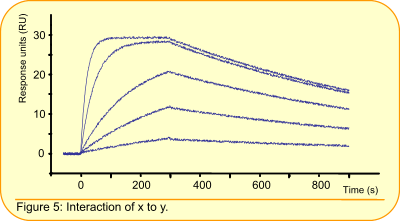What is wrong with this sensorgram?

A: the axis legends are missing
B: there are no replicates
C: the concentration range is too narrow
D: not all curves go to steady state

Question 6: Sensorgram issuesWhat is wrong with this sensorgram?

A: the axis legends are missing
B: there are no replicates
C: the concentration range is too narrow
D: not all curves go to steady state

Question 7: Before fitting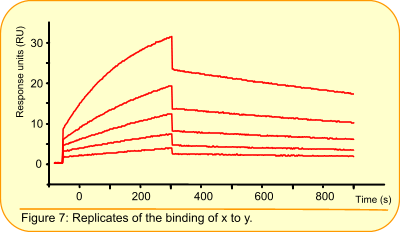What should be solved first before fitting?

A: association time should be longer
B: these are not exponentials: perform a better experiment
C: dissociation time should be longer
D: match flow and analyte buffer

Question 8: Equilibrium analysis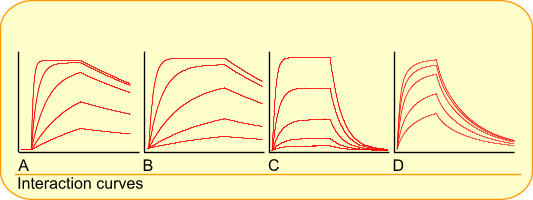Which sensorgram can you use for equilibrium analysis?

A     B     C     D

Question 9: Sensorgram optimizationWhat shoud be optimized before fitting?

A: association time longer
B: longer dissociation time
C: user higher analyte concentrations
D: use lower analyte concentrations

Question 10: Fastest dissociation rate constant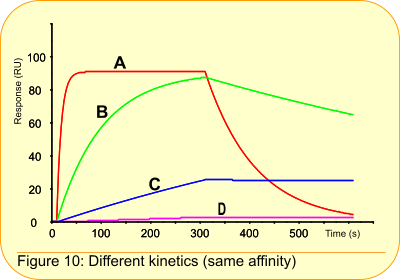Which curve has the fastest dissociation rate constant?

A: curve A
B: curve B
C: you should know the analyte concentration
D: you should know the Rmax of the system

Question 11: Fastest association rate constantWhich curve has the fastest association rate constant?
Hint: What determines the association rate.

A: curve A
B: curve B
C: you should know the analyte concentration
D: you should know the Rmax of the system

Question 12: Fastes association rate constantWhich curve has the fastest association rate constant?

A: curve A
B: they are all the same
C: curve D
D: C is higher than D

Question 13: Highest equilibrium constant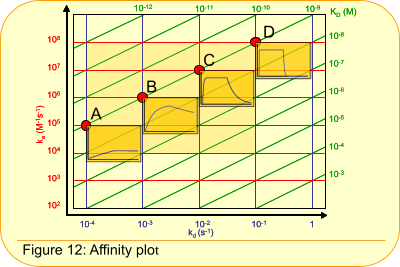Which curve has the highest equilibrium constant?

A: curve A
B: they are all the same
C: curve D
D: C is higher than D

Question 14: Fitting report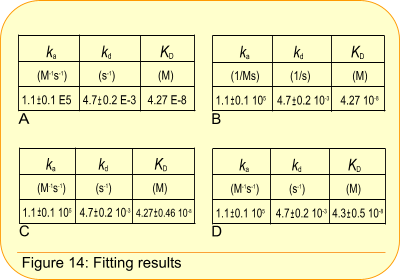Which fitting result should you report?

A     B     C     D

Question 15: Global parameters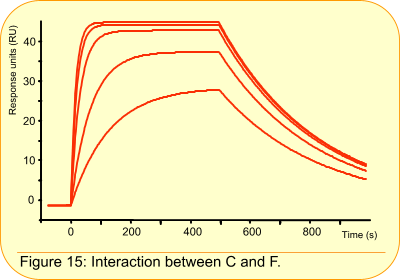Which parameters should be globally fitted?

A: ka, kd, KD, Rmax
B: ka, kd, Rmax
C: ka, kd, Rmax, RI
D: Rmax, RI, Chi2

Question 16: Sensorgram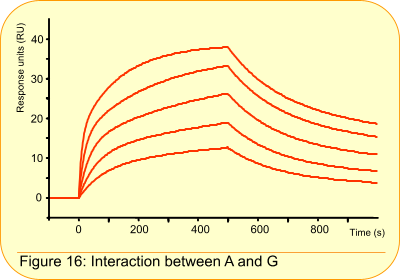What can you do when you have this sensorgram?

A: check analyte for purity
B: reverse the ligand and analyte
C: A + B
D: make the flow faster

Question 17: Sensorgram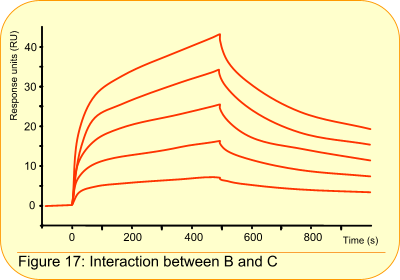What can you do when you have this sensorgram?

A: check the analyte for purity
B: reverse the analyte and ligand
C: try another immobilisation technique
D: A + B + C

Question 18: Sensorgram optimisationWhat can you do to optimize this interaction?

 1: lower ligand concentration 2: match buffers better 3: equilibrate better 4: use higher flow rate

A: 1 + 2
B: 3 + 4
C: 1 + 2 + 3
D: 2 + 3 + 4

Question 19: Ligand immobilisation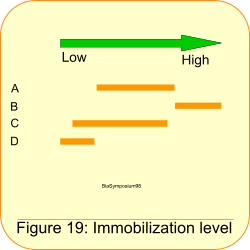How much ligand should you immobilize for analyte concentration measurements?

A     B     C     D

Question 20: Ligand immobilization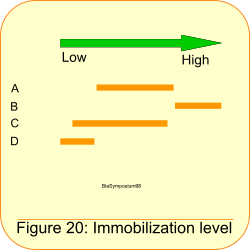How much ligand should you immobilize for kinetic analysis?

A     B     C     D

Question 21: Equilibrium curves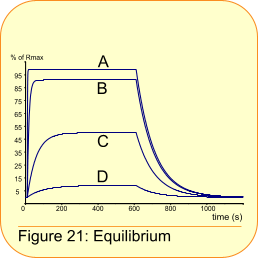Which curve is in equilibrium (steady state)?

A: curve A
B: curve B
C: curve C
D: all four curves

Question 22: Ligand saturation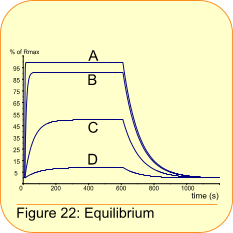Which curve is saturating the ligand?

A: curve A
B: curve B
C: curve C
D: all four curves

Question 23: KD concentrationWhich curve has an analyte concentration comparable to the KD?

A: curve A
B: curve B
C: curve C
D: all four curves

Question 24: sensorgram optimisationWhen you have this fit as a result. What can you do?
Hint: go for the best result.

 1: lower ligand concentration 2: match buffers better 3: check the ligand for purity 4: use higher flow rate

A: 1 + 2
B: 1 + 3
C: 2 + 4
D: 1 + 3 + 4

Question 25: Plausible values?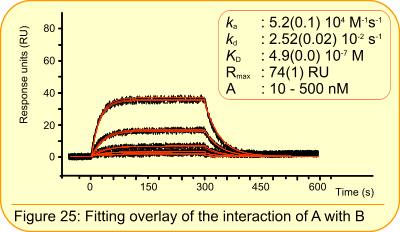Are the values given in the inset plausible with this sensorgram?
Hint: look at the curve spacing.

A: yes
B: no, dissociation looks faster
C: no, dissociations looks slower
D: no, Rmax is too high

Question 26: Change in fitting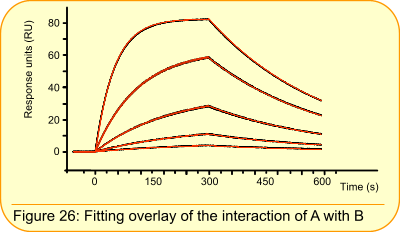What do you want to change if you see this fitting?

A: nothing, this looks fine
B: make association time longer
C: make dissociation time longer
D: use higher analyte concentration

Question 27: Meaningful results

To calculate (fit) meaningful results you need curves

A: which go to Rmax
B: which go to steady state
C: which have curvature
D: with a low response

Question 28: Rmax dependency

What can you tell about the Rmax?

A: it is dependent on the ka and kd of an interaction
B: it is dependent on the surface capacity and the molecular weight of ligand and analyte
C: it is dependent on the analyte concentration
D: B + C

Question 29: Sensorgram issues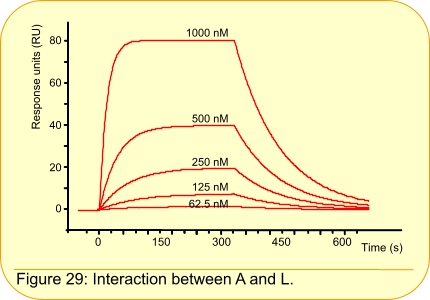A: the analyte concentration range is not wide enough
B: the response is not following exponential kinetics
C: there is mass transport limitation
D: this looks a fine sensorgram

Question 30: Publication requirements

The minimal requirements in a publication are:

A: sensorgram + fit overlay + kinetic values
B: sensorgrams (replicates) + fit overlay + kinetic values
C: table with kinetic values and representative sensorgram
D: full method used in the experiments + B